# Convolutional Neural Networks Cheatsheet

Learn more about convolutional neural networks from these resources from Stanford University to assist you on your journey through our Kambria Code Challenge: Quiz 02. Top-scoring developers will have an opportunity to have their resume and profile sent to Kambria’s corporate partners for career opportunities, so be sure to study hard, and you’ll have the opportunity to take home some exceptional prizes!

## Overview – Convolutional Neural Networks

Architecture of a traditional CNN ― Convolutional neural networks, also known as CNNs, are a specific type of neural network generally composed of the following layers: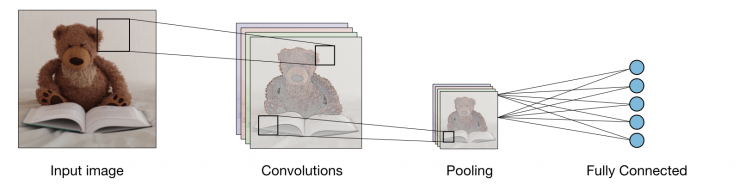The convolution layer and the pooling layer can be fine-tuned with respect to hyperparameters that are described in the next sections.

## Types of layers

Convolution layer (CONV) ― The convolution layer (CONV) uses filters that perform convolution operations as it is scanning the input with respect to its dimensions. Its hyperparameters include the filter size and stride .  The resulting output is called a feature map or activation map.

Pooling (POOL) ― The pooling layer (POOL) is a downsampling operation, typically applied after a convolution layer, which does some spatial invariance. In particular, max and average pooling are special kinds of pooling where the maximum and average value is taken, respectively.

 Type Max pooling Average pooling Purpose Each pooling operation selects the maximum value of the current view Each pooling operation averages the values of the current view Illustration
Comments • Preserves detected features • Most commonly used • Downsamples feature map • Used in LeNet

Fully Connected (FC) ― The fully connected layer (FC) operates on a flattened input where each input is connected to all neurons. If present, FC layers are usually found towards the end of CNN architectures and can be used to optimize objectives such as class scores.## Filter hyperparameters

The convolution layer contains filters for which it is important to know the meaning behind its hyperparameters.

Dimensions of a filter ― A filter of size applied to an input containing channels is a volume that performs convolutions on an input of size and produces an output feature map (also called activation map) of size .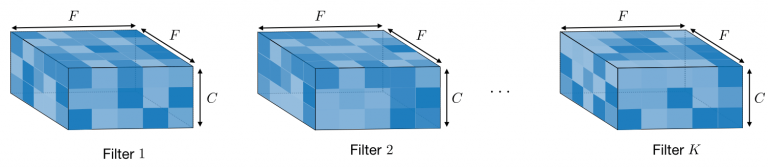Remark: the application of filters of size results in an output feature map of size .

Stride ― For a convolutional or a pooling operation, the stride  denotes the number of pixels by which the window moves after each operation.Zero-padding ― Zero-padding denotes the process of adding  zeroes to each side of the boundaries of the input. This value can either be manually specified or automatically set through one of the three modes detailed below:

 Mode Valid Same Full Value Illustration
Purpose • No padding • Drops last convolution if dimensions do not match • Padding such that feature map size has size • Output size is mathematically convenient • Also called ‘half’ padding • Maximum padding such that end convolutions are applied on the limits of the input • Filter ‘sees’ the input end-to-end

## Tuning hyperparameters

Parameter compatibility in convolution layer ― By noting the length of the input volume size, the length of the filter, the amount of zero padding, the stride, then the output size  of the feature map along that dimension is given by: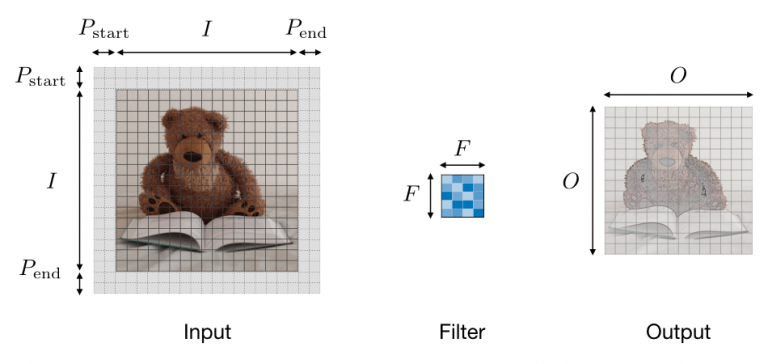Remark: often times, , in which case we can replace by  in the formula above.

Understanding the complexity of the model ― In order to assess the complexity of a model, it is often useful to determine the number of parameters that its architecture will have. In a given layer of a convolutional neural network, it is done as follows:

 CONV POOL FC Illustration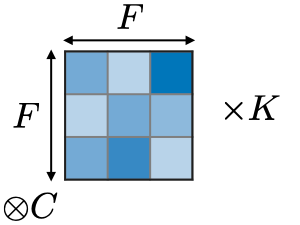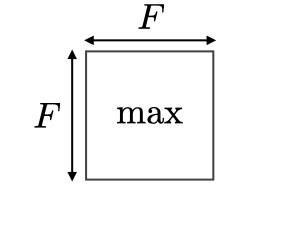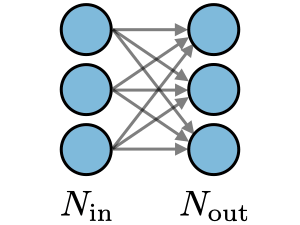Input size Output size Number of parameters 0 Remarks • One bias parameter per filter • In most cases, • A common choice for is • Pooling operation done channel-wise • In most cases, • Input is flattened • One bias parameter per neuron • The number of FC neurons is free of structural constraints

Receptive field ― The receptive field at layer  is the area denoted of the input that each pixel of the -th activation map can ‘see’. By calling the filter size of layer and the stride value of layer  and with the convention , the receptive field at layer  can be computed with the formula:

In the example below, we have and , which gives .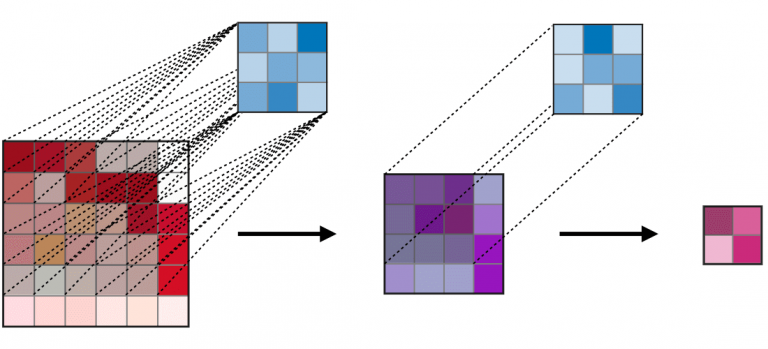## Commonly used activation functions

Rectified Linear Unit ― The rectified linear unit layer (ReLU) is an activation function  that is used on all elements of the volume. It aims at introducing non-linearities to the network. Its variants are summarized in the table below:

 ReLU Leaky ReLU ELU with with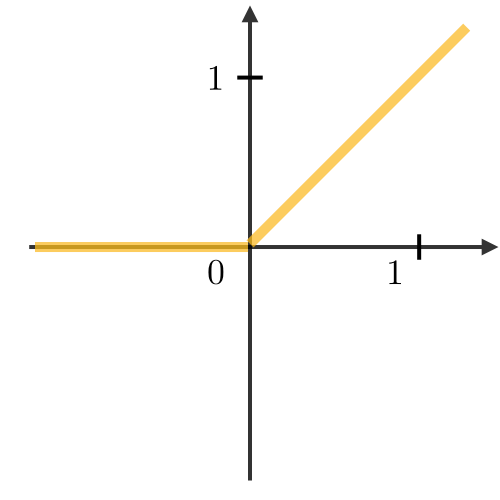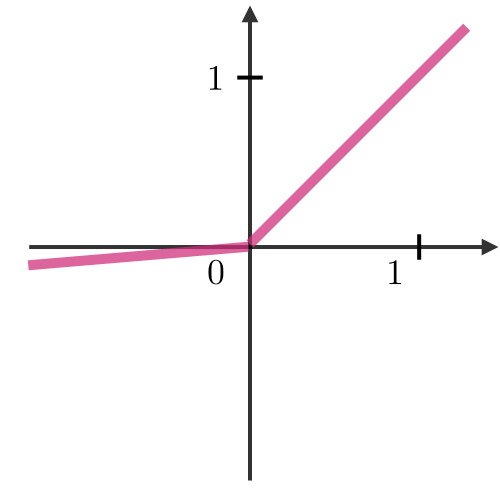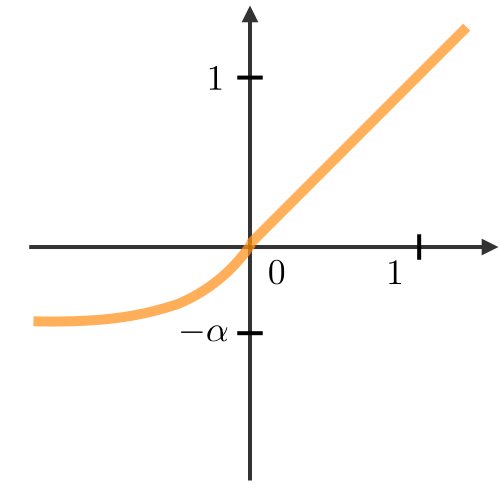• Non-linearity complexities biologically interpretable • Addresses dying ReLU issue for negative values • Differentiable everywhere

Softmax ― The softmax step can be seen as a generalized logistic function that takes as input a vector of scores and outputs a vector of output probability  through a softmax function at the end of the architecture. It is defined as follows:

## Object detection

Types of models ― There are 3 main types of object recognition algorithms for which the nature of what is predicted is different. They are described in the table below:

 Image classification Classification w. localization Detection• Classifies a picture • Predicts the probability of the object • Detects an object in a picture • Predicts the probability of the object and where it is located • Detects up to several objects in a picture • Predicts probabilities of objects and where they are located Traditional CNN Simplified YOLO, R-CNN YOLO, R-CNN

Detection ― In the context of object detection, different methods are used depending on whether we just want to locate the object or detect a more complex shape in the image. The two main ones are summed up in the table below:

 Bounding box detection Landmark detection • Detects the part of the image where the object is located • Detects a shape or characteristics of an object (e.g. eyes) • More granularBox of center , height and width Reference points

Intersection over Union ― Intersection over Union, also known as , is a function that quantifies how correctly positioned a predicted bounding box is over the actual bounding box . It is defined as:

Remark: we always have . By convention, a predicted bounding box is considered as being reasonably good if .Anchor boxes ― Anchor boxing is a technique used to predict overlapping bounding boxes. In practice, the network is allowed to predict more than one box simultaneously, where each box prediction is constrained to have a given set of geometrical properties. For instance, the first prediction can potentially be a rectangular box of a given form, while the second will be another rectangular box of a different geometrical form.

Non-max suppression ― The non-max suppression technique aims at removing duplicate overlapping bounding boxes of the same object by selecting the most representative ones. After having removed all boxes having a probability prediction lower than 0.6, the following steps are repeated while there are boxes remaining:

For a given class,
• Step 1: Pick the box with the largest prediction probability.
• Step 2: Discard any box having an  with the previous box.YOLO ― You Only Look Once (YOLO) is an object detection algorithm that performs the following steps:

• Step 1: Divide the input image into a  grid.
• Step 2: For each grid cell, run a CNN that predicts  of the following form:

where is the probability of detecting an object, are the properties of the detected bouding box, is a one-hot representation of which of the classes were detected, and  is the number of anchor boxes.
• Step 3: Run the non-max suppression algorithm to remove any potential duplicate overlapping bounding boxes.Remark: when , then the network does not detect any object. In that case, the corresponding predictions  have to be ignored.

R-CNN ― Region with Convolutional Neural Networks (R-CNN) is an object detection algorithm that first segments the image to find potential relevant bounding boxes and then run the detection algorithm to find most probable objects in those bounding boxes.Remark: although the original algorithm is computationally expensive and slow, newer architectures enabled the algorithm to run faster, such as Fast R-CNN and Faster R-CNN.

## Face verification and recognition

Types of models ― Two main types of model are summed up in the table below:

 Face verification Face recognition • Is this the correct person? • One-to-one lookup • Is this one of the  persons in the database? • One-to-many lookupOne Shot Learning ― One Shot Learning is a face verification algorithm that uses a limited training set to learn a similarity function that quantifies how different two given images are. The similarity function applied to two images is often noted .

Siamese Network ― Siamese Networks aim at learning how to encode images to then quantify how different two images are. For a given input image , the encoded output is often noted as .

Triplet loss ― The triplet loss is a loss function computed on the embedding representation of a triplet of images (anchor), (positive) and (negative). The anchor and the positive example belong to a same class, while the negative example to another one. By calling  the margin parameter, this loss is defined as follows: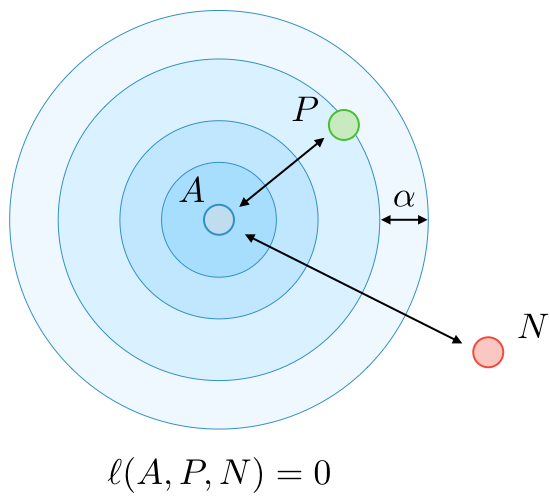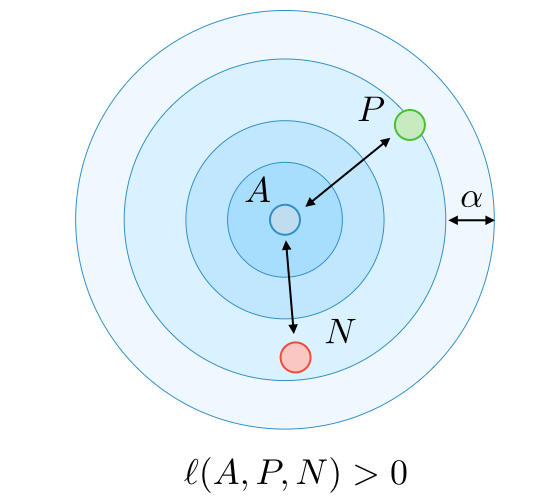## Neural style transfer

Motivation ― The goal of neural style transfer is to generate an image based on a given content and a given style .Activation ― In a given layer , the activation is noted and is of dimensions

Content cost function ― The content cost function is used to determine how the generated image differs from the original content image . It is defined as follows:

Style matrix ― The style matrix of a given layer is a Gram matrix where each of its elements quantifies how correlated the channels  and are. It is defined with respect to activations  as follows:

Remark: the style matrix for the style image and the generated image are noted and  respectively.

Style cost function ― The style cost function is used to determine how the generated image differs from the style . It is defined as follows:

Overall cost function ― The overall cost function is defined as being a combination of the content and style cost functions, weighted by parameters  as follows:

Remark: a higher value of will make the model care more about the content while a higher value of  will make it care more about the style.

## Architectures using computational tricks

Generative Adversarial Network ― Generative adversarial networks, also known as GANs, are composed of a generative and a discriminative model, where the generative model aims at generating the most truthful output that will be fed into the discriminative which aims at differentiating the generated and true image.Remark: use cases using variants of GANs include text to image, music generation and synthesis.

ResNet ― The Residual Network architecture (also called ResNet) uses residual blocks with a high number of layers meant to decrease the training error. The residual block has the following characterizing equation:

Inception Network ― This architecture uses inception modules and aims at giving a try at different convolutions in order to increase its performance through features diversification. In particular, it uses the  convolution trick to limit the computational burden.

To learn more, or to join the Kambria Code Challenge: Quiz 02, be sure to read the official announcement, including information on how you can win additional prizes by signing up early (restrictions apply).  For more info, please visit: https://bit.ly/KambriaQuiz02Announcement. Thank you and good luck!

Source: Stanford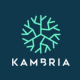Kambria is the first decentralized open innovation platform for Deep Tech (AI, Robotics, Blockchain, VR/AR…). Using our platform, anyone can collaborate in researching, developing and commercializing innovative ideas and get rewarded fairly for their contributions. Through partnerships with government agencies, top universities and leading companies, Kambria is dedicated to building a sustainable open innovation ecosystem to change the way we innovate and to accelerate advanced technology development and industry adoption. Together, let’s shape the future of technology where technology is open and contributing more to society.